Q.1
Instructions : In which answer shape question figure is present ? Question shape :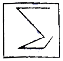Q.2
Instructions : In which answer shape question figure is present ? Question shape :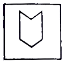Q.3
Instructions : In which answer shape question figure is present in the following ? Question shape :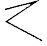Q.4
Instructions : In which answer shape question figure is present in the following ? Question shape :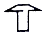Q.5
Instructions : In which answer shape question figure is present ? Question shape :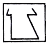Q.6
Directions : Choose that answer figure from the given options, in which question figure is present ? Question shape :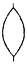Q.7
Instructions : In which answer shape given question figure is present in the following ? Question shape :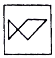Q.8
Instructions : In which answer shape given question figure is present in the following ? Question shape :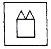Q.9
Instructions : Choose that answer shape from the given options, in which question figure is present ? Question shape :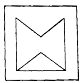Q.10
In which format given shape is present ? Question shape :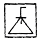Q.11
Instructions : In which answer shape question figure is present ? Question shape :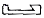Q.12
Instructions : In which answer shape question figure is present ? Question shape :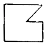Q.13
Instructions : In which answer shape question figure is present in the following ? Question shape :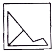Q.14
Directions : Choose that answer figure from the given answer shapes, in which question figure is present ? Question shape :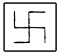Q.15
Instructions : In which answer shape question figure is present in the following ? Question shape :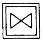Q.16
Instructions : In which answer shape question figure is present ? Question shape :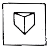Q.17
Instructions : In which answer shape question figure is present ? Question shape :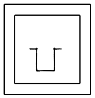Q.18
Directions : Choose that answer figure from the given answer shapes, in which question figure is present ? Question shape :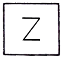Q.19
Directions : Choose that answer figure from the given answer shapes, in which question figure is present ? Question shape :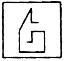Q.20
Instructions : In which answer shape question figure is present ? Question shape :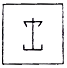Scroll